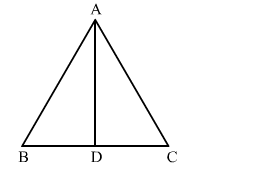# If the bisector of an angle of a triangle bisects the opposite side, then the triangle isQuestion:

If the bisector of an angle of a triangle bisects the opposite side, then the triangle is
(a) scalene
(b) equilateral
(c) isosceles
(d) right-angled

Solution:

(c) isoscelesLet AD be the angle bisector of angle A in triangle ABC.
Applying angle bisector theorem, we get:

$\frac{A B}{A C}=\frac{B D}{D C}$

It is given that AD bisects BC.
Therefore, BD = DC

$\Rightarrow \frac{A B}{A C}=1$

$\Rightarrow A B=A C$

Therefore, the triangle is isosceles.